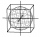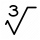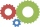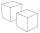# Equation + cube - math problems

#### Number of problems found: 21

• Annual growthThe population has grown from 25,000 to 33,600 in 10 years. Calculate what was the average annual population growth in%?
• The poolThe cube-shaped pool has 140 cubic meters of water. Determine the dimensions of the bottom if the depth of the water is 200 cm and one dimension of the base is 3 m greater than the other. What are the dimensions of the pool bottom?
• Derivative problemThe sum of two numbers is 12. Find these numbers if: a) The sum of their third powers is minimal. b) The product of one with the cube of the other is maximal. c) Both are positive and the product of one with the other power of the other is maximal.
• Magnified cubeIf the lengths of the cube's edges are extended by 5 cm, its volume will increase by 485 cm3. Determine the surface of both the original and the magnified cube.
• Cuboid and ratioFind the dimensions of a cuboid having a volume of 810 cm3 if the lengths of its edges coming from the same vertex are in ratio 2: 3: 5
• Water containerThe cube-shaped container is filled to two-thirds of its height. If we pour 18 liters, it will be filled to three-fifths of the height. What is the volume of the whole container?
• Container with waterThe cube-shaped container is filled with water to half its height. If we add 20 liters of water, the container will be filled to three-quarters of its height. What is the volume of the whole container?
• One third powerWhich equation justifies why ten to the one-third power equals the cube root of ten?
• Six speedsA drilling machine is to have 6 speeds ranging from 50 to 750 revolutions per minute. If the speed forms a geometric progression, determine their values.
• Three members GPThe sum of three numbers in GP (geometric progression) is 21 and the sum of their squares is 189. Find the numbers.
• Two cubesThe surfaces of two cubes, one of which has an edge of 22 cm longer than the second are differ by 19272 cm2. Calculate the edge length of both cubes.
• Endless lego setThe endless lego set contains only 6, 9, 20 kilograms blocks that can no longer be polished or broken. The workers took them to the gym and immediately started building different buildings. And of course, they wrote down how much the building weighed. The4 m long ladder touches the cube 1mx1m at the wall. How high reach on the wall?
• Body diagonalThe cuboid has a volume of 32 cm3. Its side surface area is double as one of the square bases. What is the length of the body diagonal?
• Rectangle poolFind dimensions of an open pool with a square bottom with a capacity of 32 m3 to have painted/bricked walls with the least amount of material.
• Z9-I-4Kate thought a five-digit integer. She wrote the sum of this number and its half at the first line to the workbook. On the second line wrote a total of this number and its one fifth. On the third row, she wrote a sum of this number and its one nines. Fina
• Tetrahedral prismThe height of a regular tetrahedral prism is three times greater than the length of the base edge. Calculate the length of the base edge, if you know that the prism volume is 2187 cm3.
• Variations 3rd classFrom how many elements we can create 13,800 variations 3rd class without repeating?
• Cubes - diffSecond cubes edge is 2 cm longer than the edge of the first cube. Volume difference blocks is 728 cm3. Calculate the sizes of the edges of the two dice.
• CubesSurfaces of cubes, one of which has an edge of 48 cm shorter than the other, differ by 36288 dm2. Determine the length of the edges of this cubes.

Do you have an exciting math question or word problem that you can't solve? Ask a question or post a math problem, and we can try to solve it.

We will send a solution to your e-mail address. Solved examples are also published here. Please enter the e-mail correctly and check whether you don't have a full mailbox.

Do you have a linear equation or system of equations and looking for its solution? Or do you have a quadratic equation? Equations Math problems. Cube Problems.